# Another answer I got was 62.78 Amps but that is also incorrect. 4. 0/1 pointsI Previous...

Another answer I got was 62.78 Amps but that is also incorrect.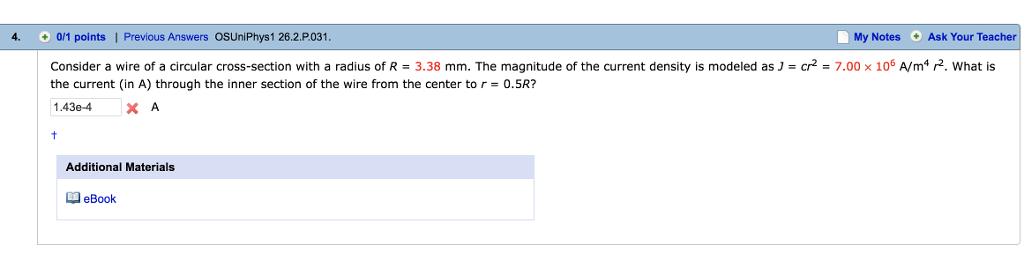4. 0/1 pointsI Previous Answers OSUniPhys1 26.2.P031 My Notes Ask Your Teacher Consider a wire of a circular cross-section with a radius of R-3.38 mm. The magnitude of the current density is modeled as J-cr2 = 7.00 × 106 A/m4戸. what is the current (in A) through the inner section of the wire from the center to r 0.5R? 1.43e-4 X A Additional Materials eBook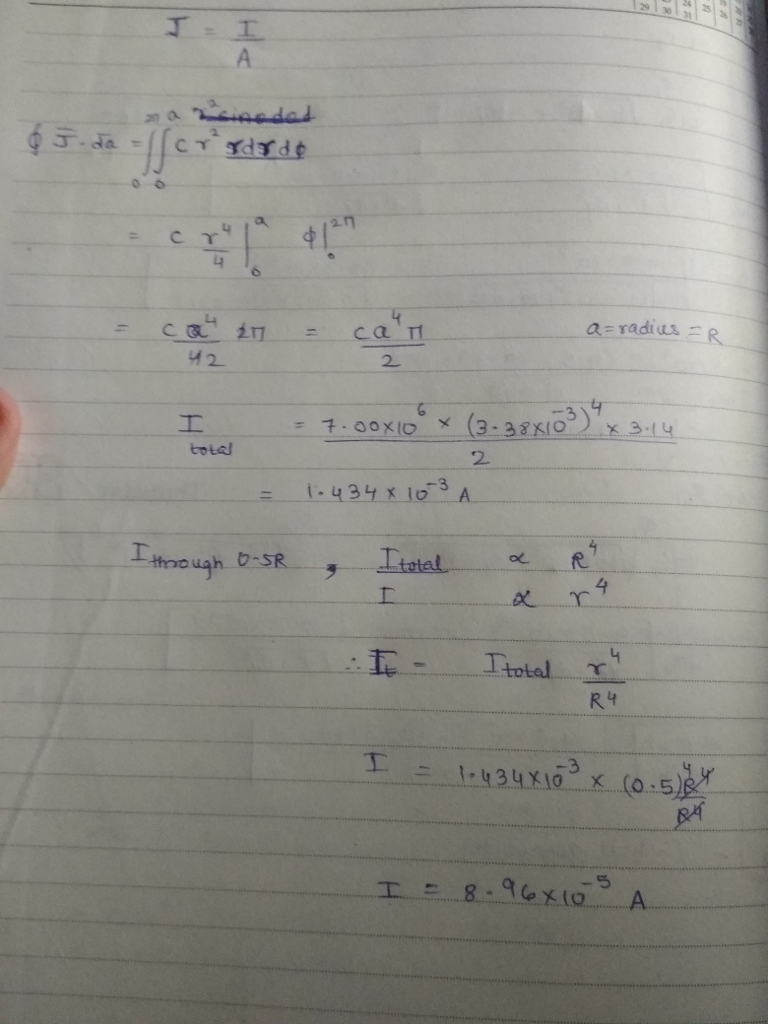##### Add Answer to: Another answer I got was 62.78 Amps but that is also incorrect. 4. 0/1 pointsI Previous...
Similar Homework Help Questions
• ### as ) = cr2 Consider a wire of a circular cross-section with a radius of R...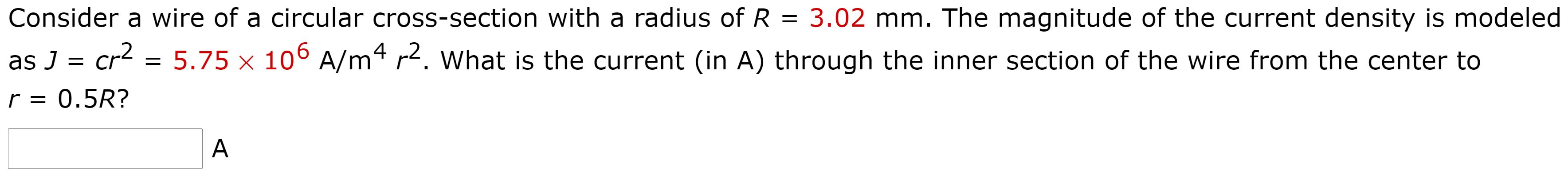as ) = cr2 Consider a wire of a circular cross-section with a radius of R 3.02 mm. The magnitude of the current density is modeled 5.75 X 106 A/m4 r2. What is the current (in A) through the inner section of the wire from the center to 0.5R? r = A

• ### consider a wire or a circular cross-section with a radius of R = 3.46 mm. The...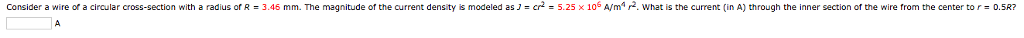consider a wire or a circular cross-section with a radius of R = 3.46 mm. The magnitude of the current density is modeled as ] = c,2-5.25 × 106 A/m4 r2. what is the current (in A) through the inner section of the wire from the center to r-0.5R?

• ### 3. 0/2 points Previous Answers OSUN Phys1 20.2 P001. My Notes -3.75 X 100 A/ m...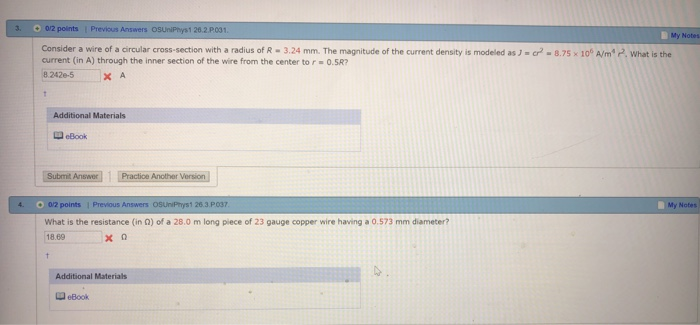3. 0/2 points Previous Answers OSUN Phys1 20.2 P001. My Notes -3.75 X 100 A/ m . What is the Consider a wire of a circular cross-section with a radius of R - 3.24 mm. The magnitude of the current density is modeled as J = current (in A) through the inner section of the wire from the center to r=0.5R? 8.242e-5 XA Additional Materials eBook Submit Answer 1 Practice Another Version 4. My Notes 02 points 1 Previous Answers...

• ### Will like for answer! 10. + 0/1 points Previous Answers OSUniPhys1 29.6.P.057. My Notes A toroid...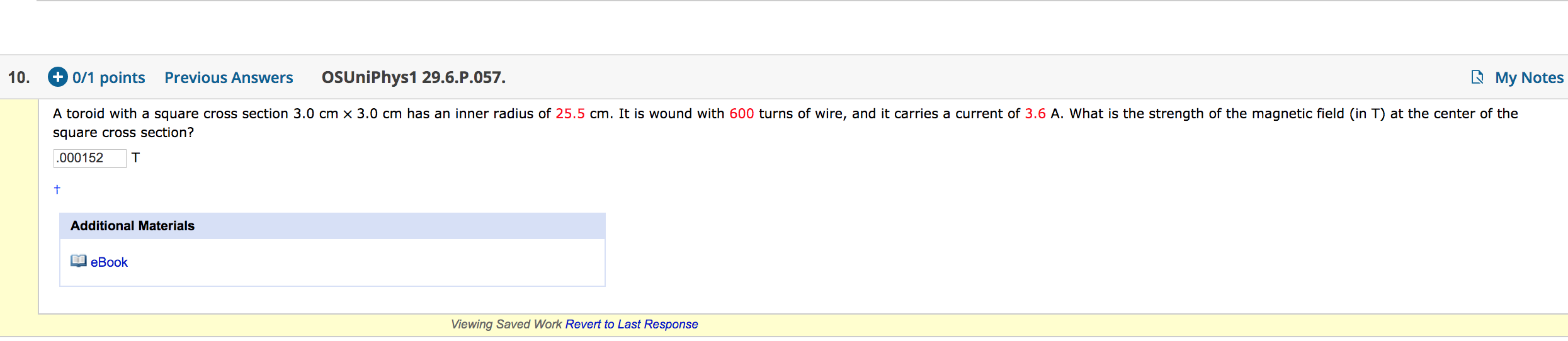Will like for answer! 10. + 0/1 points Previous Answers OSUniPhys1 29.6.P.057. My Notes A toroid with a square cross section 3.0 cm x 3.0 cm has an inner radius of 25.5 cm. It is wound with 600 turns of wire, and it carries a current of 3.6 A. What is the strength of the magnetic field (in T) at the center of the square cross section? .000152 T Additional Materials eBook Viewing Saved Work Revert to Last Response

• ### 5. [0/1 Points] DETAILS PREVIOUS ANSWERS OSUNIPHYS1 28.4.WA.018. MY NOTES ASK YOUR TEACHER PRACTICE ANOTHER Two...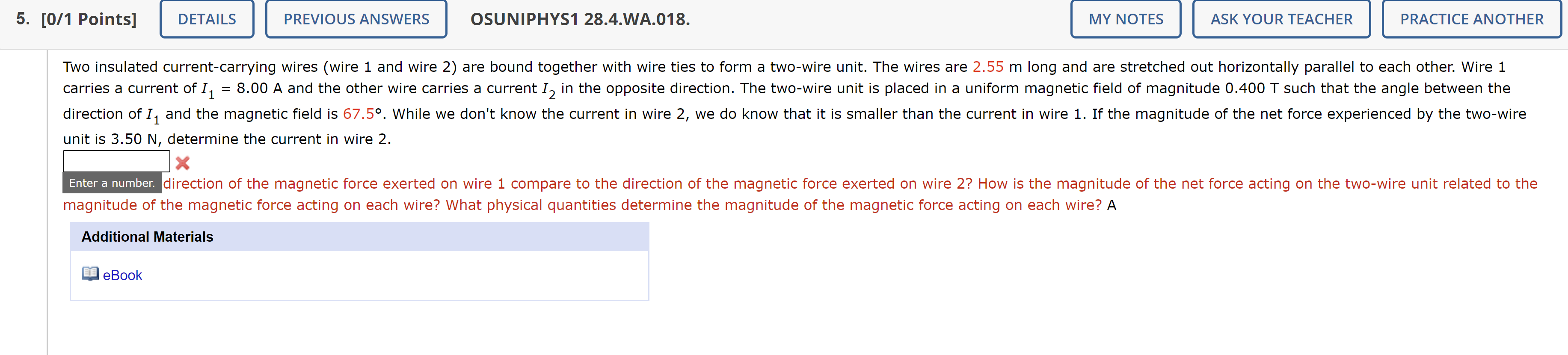5. [0/1 Points] DETAILS PREVIOUS ANSWERS OSUNIPHYS1 28.4.WA.018. MY NOTES ASK YOUR TEACHER PRACTICE ANOTHER Two insulated current-carrying wires (wire 1 and wire 2) are bound together with wire ties to form a two-wire unit. The wires are 2.55 m long and are stretched out horizontally parallel to each other. Wire 1 carries a current of I1 = 8.00 A and the other wire carries a current Iz in the opposite direction. The two-wire unit is placed in a uniform...

• ### eriais eBook Submit Answer -/1 Points] DETAILS SERPSET9 30.P.044. MY NOTES ASK YOUR TEACHER PRACTICE ANOTHER...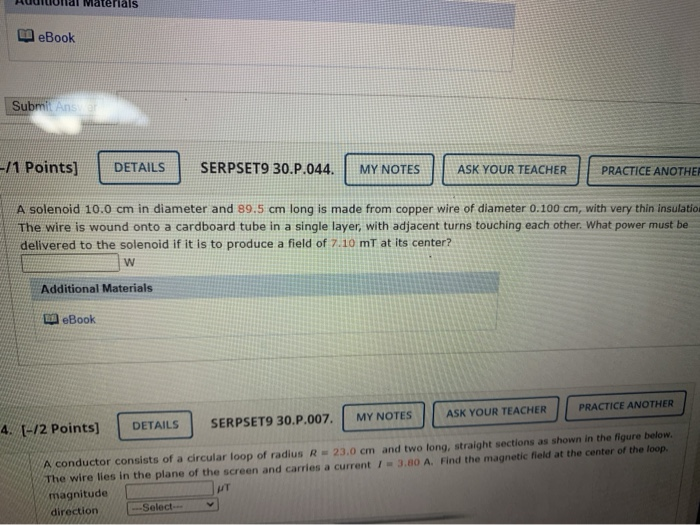eriais eBook Submit Answer -/1 Points] DETAILS SERPSET9 30.P.044. MY NOTES ASK YOUR TEACHER PRACTICE ANOTHER A solenoid 10.0 cm in diameter and 89.5 cm long is made from copper wire of diameter 0.100 cm, with very thin insulatio The wire is wound onto a cardboard tube in a single layer, with adjacent turns touching each other. What power must be delivered to the solenoid if it is to produce a field of 7.10 mt at its center? w Additional...

Free Homework App